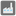• # ErrorTooLongToProcess

## Question related to missionPower Plants

Hi,

I got the above error when I checked my code. Basically my program runs as follows: 1. obtain a matrix of distances between cities 2. given a permutation of cities of length equal to the number of power plants, see if every city is covered. It seems the first step is taking a huge amount of time, when the cities are many. But I don't think it's possible to improve this, as the distance records seem indispensable to me.

Here are my codes for reference (sorry that there are some long lines):

```from itertools import permutations
def power_plants(network:set, ranges:list):
def dist(a:str, b:str, network = network):
if a == b: return 0
autre = lambda x: x[not x.index(a)]
return 1 + min([dist(autre(c),b,
network=[d for d in network if a not in d]) for c in network if a in c],default = 50)

cities = list(set(s for s in format(network) if s.isalpha()))
m = len(cities)
graph = [[dist(s,t) for t in cities] for s in cities]
form = lambda S: {cities[S[i]]:ranges[i] for i in range(len(ranges))}
for S in permutations(range(m), len(ranges)):
if all(any(graph[c][S[i]]<= ranges[i] for i in range(len(ranges))) for c in range(m)): return form(S)
return None
```17